# Optical window in biological tissue

﻿
Optical window in biological tissue

The Optical window  (also known as therapeutic window) defines the range of wavelengths where light has its maximum depth of penetration in tissue. Within the NIR window, scattering is the most dominant light-tissue interaction, and therefore the propagating light becomes diffuse rapidly. Since scattering increases the distance travelled by photons within tissue, the probability of photon absorption also increases. Because scattering has weak dependence on wavelength, the optical window is primarily limited by absorption, due to either blood at short wavelengths or water at long wavelengths.

## Absorption properties of tissue components

The absorption coefficient ($\mu_{a} \,$) is defined as the probability of photon absorption in tissue per unit path length . Different tissue components have different$\mu_a \,$ values. Moreover,$\mu_a \,$ is a function of wavelength. Below are discussed the absorption properties of the most important chromophores in tissue. Also, molar extinction coefficient ($\varepsilon \,$) is another parameter that is often used to describe photon absorption in tissue. By multiplying e by the molar concentration and by ln(10), one can convert$\varepsilon \,$ to$\mu_a \,$.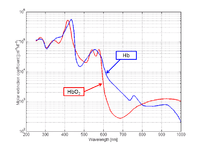Figure 1: The molar extinction coefficients of HbO2 and Hb .

Blood: Blood consists of two different types of hemoglobin: oxyhemoglobin ($HbO_2 \,$) is bound to oxygen, while deoxyhemoglobin ($Hb \,$) is unbound to oxygen. These two different types of hemoglobin exhibit different absorption spectra that are normally represented in terms of molar extinction coefficients, as shown in Figure 1. The molar extinction coefficient of Hb has its highest absorption peak at 420 nm and a second peak at 580 nm. Its spectrum then gradually decreases as light wavelength increases. On the other hand,$HbO_2 \,$ shows its highest absorption peak at 410 nm, and two secondary peaks at 550 nm and 600 nm. As light wavelengths passes 600 nm,$HbO_2 \,$ absorption decays much faster than Hb absorption. The points where the molar extinction coefficient spectra of$Hb \,$ and$HbO_2 \,$ intersect are called isosbestic points.

By using two different wavelengths, it is possible to calculate the concentrations of oxyhemoglobin ($C_{HbO2} \,$) and deoxyhemoglobin ($C_{Hb}\,$) as shown in the following equations:$\mu_a(\lambda_1) = \ln(10)\varepsilon_{HbO2}(\lambda_1)C_{HbO2}+\ln(10)\varepsilon_{Hb}(\lambda_1)C_{Hb} \,$$\mu_a(\lambda_2) = \ln(10)\varepsilon_{HbO2}(\lambda_2)C_{HbO2}+\ln(10)\varepsilon_{Hb}(\lambda_2)C_{Hb} \,$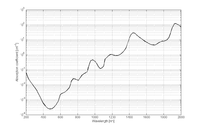Figure 2: The absorption spectrum of water .

Here,$\lambda_1 \,$ and$\lambda_2 \,$ are the two wavelengths;$\varepsilon_{HbO2} \,$ and$\varepsilon_{Hb} \,$ are the molar extinction coefficients of$HbO_2 \,$ and$Hb \,$, respectively;$C_{HbO2} \,$ and$C_{Hb} \,$ are the molar concentrations of$HbO_2 \,$ and$Hb \,$ in tissue, respectively. Oxygen saturation ($SO_2 \,$) can then be computed as$SO_2=\frac {C_{HbO2}} {C_{HbO2}+C_{Hb}}$

Water: Although water is nearly transparent in the range of visible light, it becomes absorbing over the near-infrared region. Water is a critical component since its concentration is high in human tissue. The absorption spectrum of water in the range from 250 to 1000 nm is shown in Figure 2.

Other tissue components with less significant contributions to the total absorption spectrum of tissue are melanin and fat.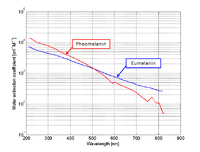Figure 3: The molar extinction coefficients of eumelanin and pheomelanin .

Melanin: Melanin is a chromophore that exists in the human epidermal layer of skin responsible for protection from harmful UV radiation. When melanocytes are stimulated by solar radiation, melanin is produced. Melanin is one of the major absorbers of light in some biological tissue (although its contribution is smaller than other components). There are two types of melanin: eumelanin which is black-brown and pheomelanin which is red-yellow. The molar extinction coefficient spectra corresponding to both types are shown in Figure 3.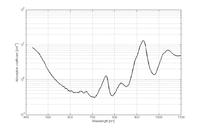Figure 4: The absorption coefficient spectrum of oil from pig lard .

Fat: Fat is one of the major components in tissue that can comprise 10-40% of tissue. Although not many mammalian fat spectra are available, Figure 4 shows an example extracted from . This plot was obtained from clear purified oil from pig lard. The detailed purification process is explained in .

## Scattering properties of tissue components

Optical scattering occurs due to mismatches in refractive index of the different tissue components, ranging from cell membranes to whole cells. Cell nuclei and mitochondria are the most important scatterers . Their dimensions range from 100 nm to 6 μm. Most of these organelles exhibit highly forward-directed scattering .

Light scattering in biological tissue is denoted by the scattering coefficient (μs), which is defined as the probability of photon scattering in tissue per unit path length .

## Effective attenuation coefficient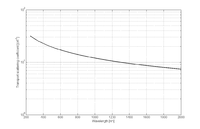Figure 5: : Transport scattering coefficient spectrum of human breast tissue .

Attenuation of light in deep biological tissue depends on the effective attenuation coefficient ($\mu_{eff} \,$), which is defined as$\mu_{eff}=\sqrt{3\mu_a(\mu_a+\mu'_s)}$

where$\mu^'_s$ is the transport scattering coefficient defined as$\mu'_s=\mu_s (1-g) \,$

where g is the anisotropy of biological tissue, which has a representative value of 0.9. Figure 5 shows a plot of transport scattering coefficient spectrum in breast tissue, which has a wavelength dependence of$\lambda \,^{-0.7}$. The effective attenuation coefficient is the dominant factor for determining light attenuation at depth$d \,$ >> 1/$\mu'_s \,$.

## Estimation of the optical window in tissue

The optical window can be computed based on the absorption coefficient spectrum or the effective attenuation coefficient spectrum. A possible criterion for selecting the NIR window is given by the FWHM of the inverse of these spectra as shown in Figure 7.

In addition to the total concentration of hemoglobin, the oxygen saturation will define the concentration of oxy and deoxyhemoglobin in tissue and so the total absorption spectrum. Depending on the type of tissue, we can consider different situations.Below, the total concentration of hemoglobin is assumed to be 2.3 mM.

Figure 6 (a): Spectra for arteries (SaO2 ≈ 98%).

Absorption coefficient: λmin = 686 nm; NIR window = (634 - 756) nm.

Effective attenuation coefficient: λmin = 690 nm; NIR window = (618 - 926) nm.

Figure 6 (b): Spectra for veins (SvO2 ≈ 60%).

Absorption coefficient: λmin = 730 nm; NIR window = (664 - 932) nm.

Effective attenuation coefficient: λmin = 730 nm; NIR window = (630 - 1328) nm.

Figure 6 (c): Spectra for breast tissue (StO2 ≈ 70%).

Absorption coefficient: λmin = 730 nm; NIR window = (656 - 916) nm.

Effective attenuation coefficient: λmin = 730 nm; NIR window = (626 - 1316) nm.

Absorption spectrum for arteries: In this case$SaO_2 \,$ ≈ 98% (arterial oxygen saturation). Then oxyhemoglobin will be dominant in the total absorption (black) and the effective attenuation (magenta) coefficient spectra, as shown in Figure 6 (a).

Absorption spectrum for veins: In this case$SvO_2 \,$ ≈ 60% (venous oxygen saturation). Then oxyhemoglobin and deoxyhemoglobin will have similar contributions to the total absorption (black) and the effective attenuation (magenta) coefficient spectra, as shown in Figure 6 (b).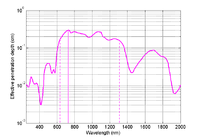Figure 7: : Effective penetration depth in breast tissue (StO2 ≈ 70%). Effective attenuation coefficient: λmin = 730 nm; NIR window = (626 - 1316) nm.

Absorption spectrum for breast tissue: To define$StO_2 \,$ (tissue oxygen saturation) it is necessary to define a distribution of arteries and veins in tissue. an arterial-venous blood volume ratio of 20%/80% can be adopted . Thus tissue oxygen saturation can be defined as$StO_2 \,$ = 0.2 x$SaO_2 \,$ + 0.8 x$SvO_2 \,$ ≈ 70%.

The total absorption (black) and the effective attenuation (magenta) coefficient spectra for breast tissue is shown in Figure 6 (c).In addition, the effective penetration depth is plotted in Figure 7.

Wikimedia Foundation. 2010.

### Look at other dictionaries:

• Near-infrared window in biological tissue — The near infrared (NIR) window (also known as optical window or therapeutic window) defines the range of wavelengths where light has its maximum depth of penetration in tissue. Within the NIR window, scattering is the most dominant light tissue… …   Wikipedia

• Optical window — Rough plot of Earth s atmospheric transmittance (or opacity) to various wavelengths of electromagnetic radiation, including visible light. The meaning of this term depends on the context: In astronomy, the optical window is the optical portion of …   Wikipedia

• Optical coherence tomography — Intervention Optical Coherence Tomography (OCT) image of a sarcoma MeSH …   Wikipedia

• Tissue engineering — Principle of tissue engineering Tissue engineering was once categorized as a sub field of bio materials, but having grown in scope and importance it can be considered as a field in its own right. It is the use of a combination of cells,… …   Wikipedia

• Optical microscope — Microscope Uses Small sample observation Notable experiments Discovery of cells Inventor Hans Lippershey Zacharias Jans …   Wikipedia

• Ceramic engineering — Simulation of the outside of the Space Shuttle as it heats up to over 1,500 °C (2,730 °F) during re entry into the Earth s atmosphere Ceramic engineering is the science and technology of creating objects from inorganic, non metallic… …   Wikipedia

• Scanning electron microscope — These pollen grains taken on an SEM show the characteristic depth of field of SEM micrographs …   Wikipedia

• Visual prosthesis — For the non functional prosthesis or glass eye see Ocular prosthesis and Craniofacial prosthesis. A visual prosthesis, often referred to as a bionic eye, is an experimental visual device intended to restore functional vision in those suffering… …   Wikipedia

• Cryoprotectant — A cryoprotectant is a substance that is used to protect biological tissue from freezing damage (damage due to ice formation). Arctic and Antarctic insects, fish, amphibians and reptiles create cryoprotectants (antifreeze compounds and antifreeze… …   Wikipedia

• Crysis 2 — Official cover art for Crysis 2 Developer(s) Crytek Frankfurt Crytek UK (multiplayer) …   Wikipedia